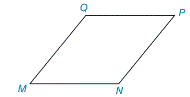Chapter 8.1, Problem 4E### Elementary Geometry for College St...

6th Edition
Daniel C. Alexander + 1 other
ISBN: 9781285195698

#### Solutions

Chapter
Section### Elementary Geometry for College St...

6th Edition
Daniel C. Alexander + 1 other
ISBN: 9781285195698
Textbook Problem
1 views

# If MNPQ is a rhombus, which formula from this section should be used to calculate its area?Exercise 4–6

To determine

To Calculate:

The area of a rhombus MNPQ.

Explanation

Calculation:

Given,

If MNPQ is a rhombus,

Rhombus has four equal sides and its diagonals bisect each other at right angles.

Here,

The rhombus of diagonal d1=MP

The rhombus of diagonal d2=NQ

From the diagram,

Here, ON=OQ=d22 and OP=OM=d12

ΔNOM,ΔPON,ΔQON and ΔMOQ are the right triangles in the rhombus.

Thus, the area of rhombus is equal to the sum of the area of right triangle ΔNOM(A1),ΔPON(A2),ΔQON(A3) and ΔMOQ(A4)

The area of rhombus (A)=A1+A2+A3+A4

For the right ΔNOM,

The area of the right ΔNOM(A1)=12ab unit2

Here, ON=a=d22 and OM=b=d12

A1=12d22d12

=d2d18

A1=d1d28

For the right ΔPON,

Area of the right ΔPON(A2)=12ab

Here, ON=a=d22 and OP=b=d12

A2=12d22d12

A2=d2d18

A2=d1d2

### Still sussing out bartleby?

Check out a sample textbook solution.

See a sample solution

#### The Solution to Your Study Problems

Bartleby provides explanations to thousands of textbook problems written by our experts, many with advanced degrees!

Get Started

#### Find more solutions based on key concepts